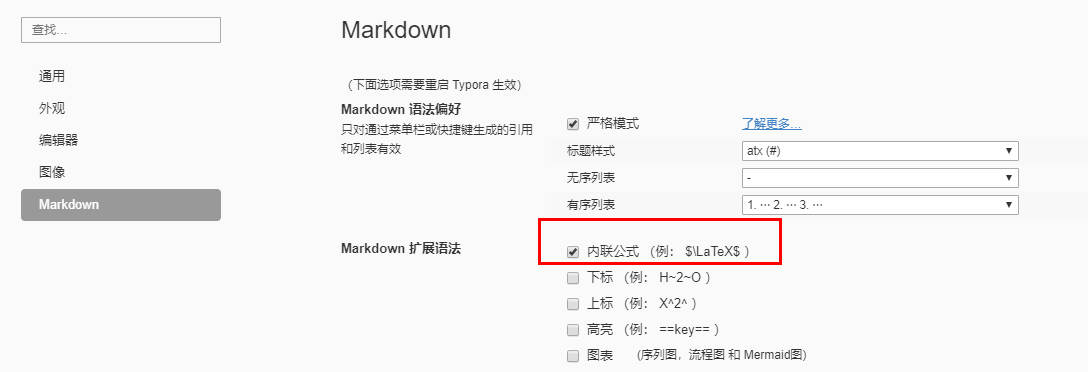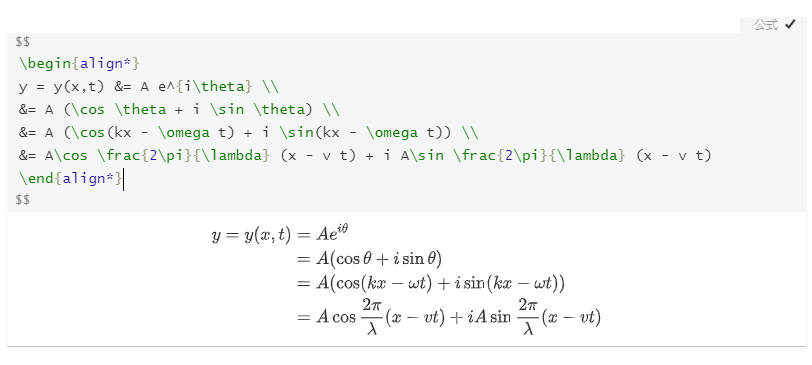# Jekyll 渲染 LaTeX 数学公式

Posted by kevin on December 24, 2019

## solution<script type="text/x-mathjax-config">
MathJax.Hub.Config({
tex2jax: {
skipTags: ['script', 'noscript', 'style', 'textarea', 'pre'],
inlineMath: [['$','$']]
}
});
</script>
<script src="https://cdn.mathjax.org/mathjax/latest/MathJax.js?config=TeX-AMS-MML_HTMLorMML" type="text/javascript"></script>


$y = \sin(\pi + \theta)$


$y = \sin(\pi + \theta)$

\begin{align*} y = y(x,t) &= A e^{i\theta} \\ &= A (\cos \theta + i \sin \theta) \\ &= A (\cos(kx - \omega t) + i \sin(kx - \omega t)) \\ &= A\cos \frac{2\pi}{\lambda} (x - v t) + i A\sin \frac{2\pi}{\lambda} (x - v t) \end{align*}

\begin{align*} y = y(x,t) &= A e^{i\theta} \\ &= A (\cos \theta + i \sin \theta) \\ &= A (\cos(kx - \omega t) + i \sin(kx - \omega t)) \\ &= A\cos \frac{2\pi}{\lambda} (x - v t) + i A\sin \frac{2\pi}{\lambda} (x - v t) \end{align*}

## reference

https://stackoverflow.com/questions/26275645/how-to-support-latex-in-github-pages